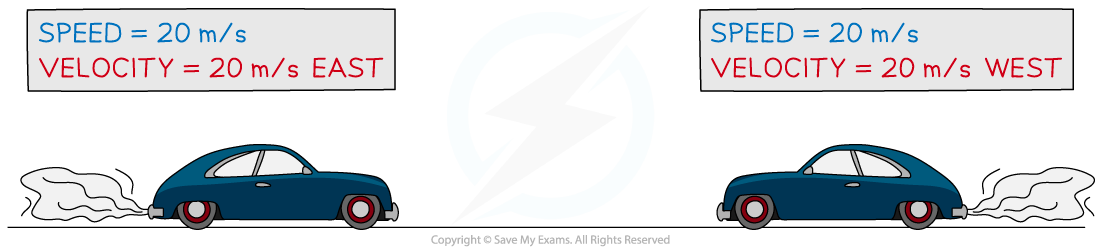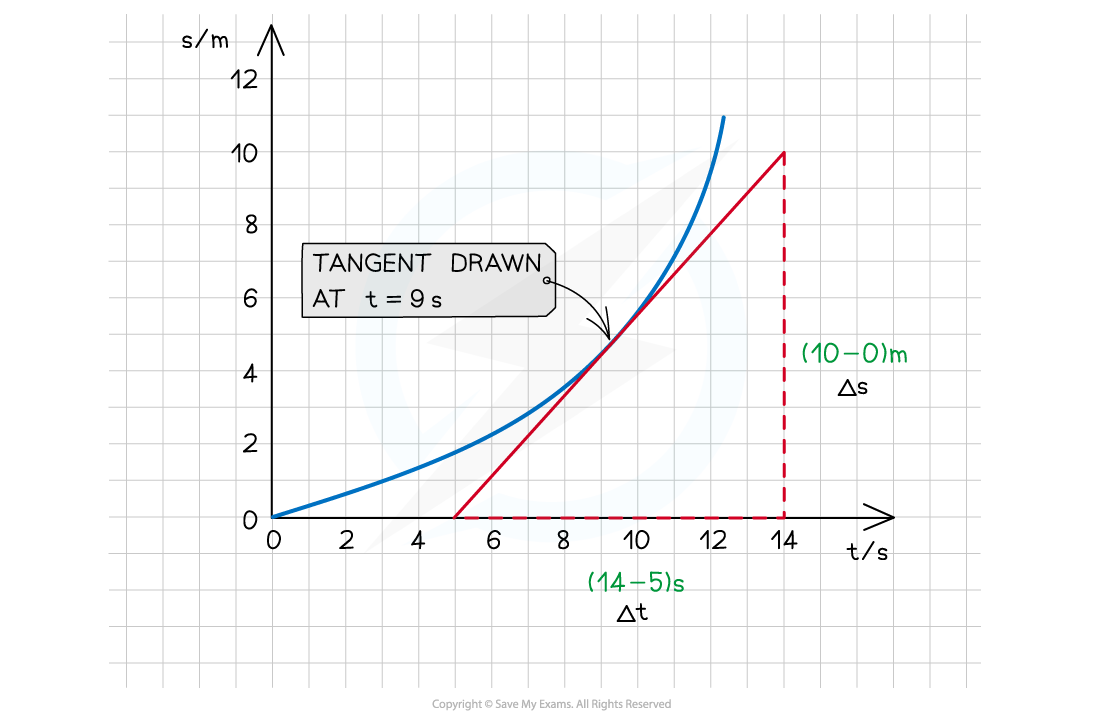# IB DP Physics: SL复习笔记2.1.2 Speed & Velocity

### Speed & Velocity

#### Speed

• The speed of an object is the distance it travels every second
• Speed is a scalar quantity
• This is because it only contains a magnitude (without a direction)
• The average speed of an object is given by the equation:• Speed is typically measured in meters per second, m/s, but can be measured in alternative units when it is more appropriate for the situation

#### Worked Example

Florence Griffith Joyner set the women’s 100 m world record in 1988, with a time of 10.49 s.Calculate her average speed during the race.

Step 1: List the known quantities

• Distance, s = 100 m
• Time, t = 10.49 s

Step 2: Write the relevant equation

• Sprinters typically speed up out of the blocks up to some maximum speed
• Because Florence’s speed changes over the race, we can calculate her average speed using the equation:

average speed = total distance ÷ time taken

Step 3: Check any unit conversions

• Check that all quantities given in the question are in standard units
• In this example, they are all in standard units

Step 4: Substitute the values for total distance and time

Average speed = 100 ÷ 10.49 = 9.53288... = 9.53 m/s

#### Velocity

• The velocity of a moving object is similar to its speed, except it also describes the object’s direction
• The speed of an object only contains a magnitude - it’s a scalar quantity
• The velocity of an object contains both magnitude and direction, e.g. ‘15 m/s south’ or ‘250 km/h on a bearing of 030°’
• Velocity is, therefore, a vector quantity because it describes both magnitude and directionThe cars in the diagram above have the same speed (a scalar quantity) but different velocities (a vector quantity). Fear not, they are in different lanes!

#### Instantaneous Speed / Velocity

• The instantaneous speed (or velocity) is the speed (or velocity) of an object at any given point in time
• This could be for an object moving at a constant velocity or accelerating
• An object accelerating is shown by a curved line on a displacement – time graph
• An accelerating object will have a changing velocity
• To find the instantaneous velocity on a displacement-time graph:
• Draw a tangent at the required time
• Calculate the gradient of that tangentThe instantaneous velocity is found by drawing a tangent on the displacement time graph

#### Average Speed / Velocity

• The average speed (or velocity) is the total distance (or displacement) divided by the total time
• To find the average velocity on a displacement-time graph, divide the total displacement (on the y-axis) by the total time (on the x-axis)
• This method can be used for both a curved or a straight line on a displacement-time graph

#### Exam Tip

When you draw a tangent to a curve, make sure it just touches the point at which you wish to calculate the gradient. The angle between the curve and the tangent line should be roughly equal on both sides of the point.